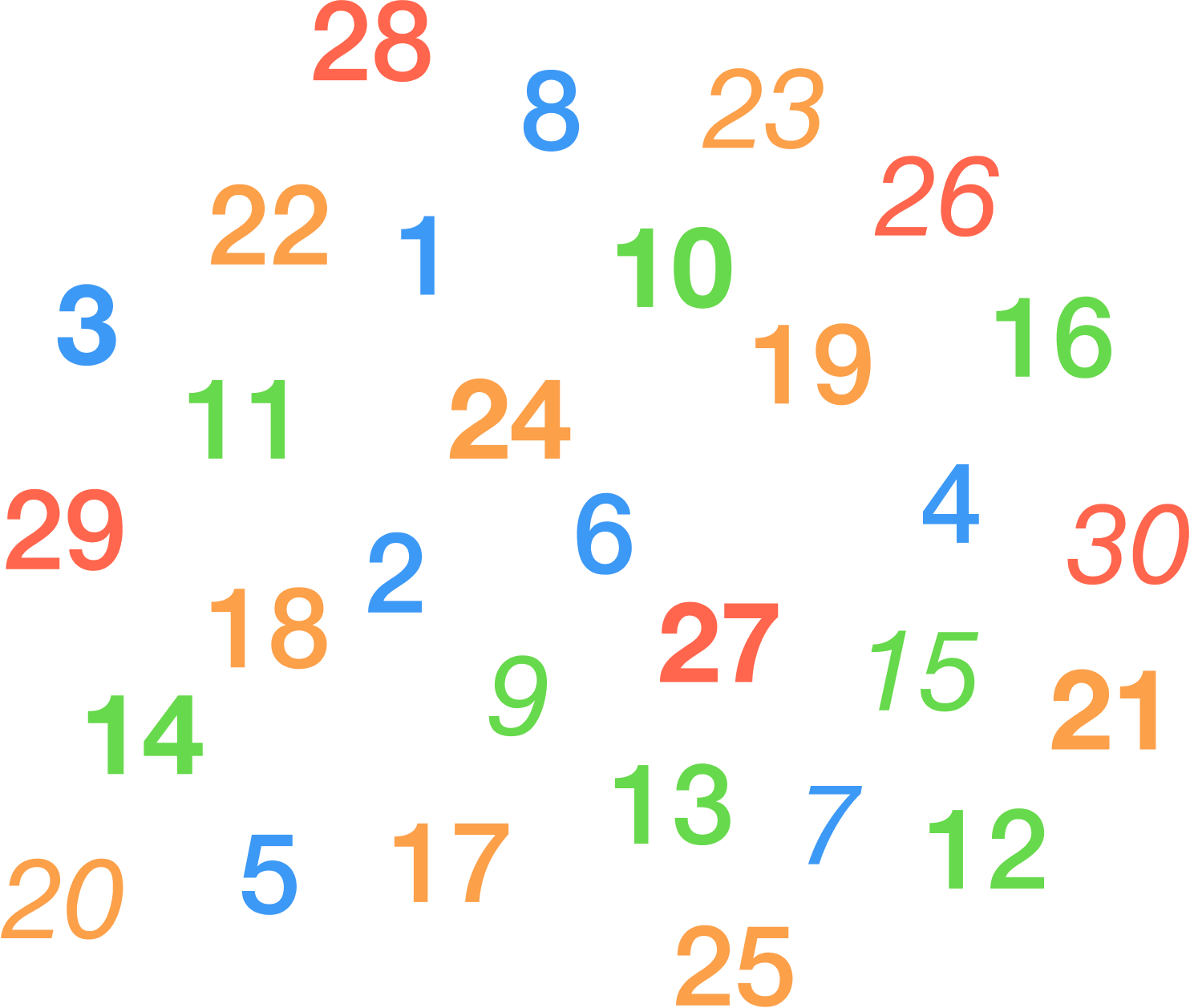# Random Probability!

A number is selected at random from the first thirty natural numbers. What is the probability that it is a multiple of EITHER $3$ or $13$?×